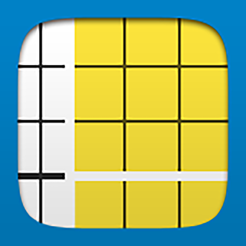# Number Pieces, by Math Learning Center

Math Learning CenterNumber Pieces helps students develop a deeper understanding of place value while building their computation skills with multi-digit numbers. Students use the pieces to represent multi-digit numbers, regroup, add, subtract, multiply, and divide.

iOS, Web Browser

### Grade Level

Elementary (1-6), Kindergarten, Pre-Kindergarten

None